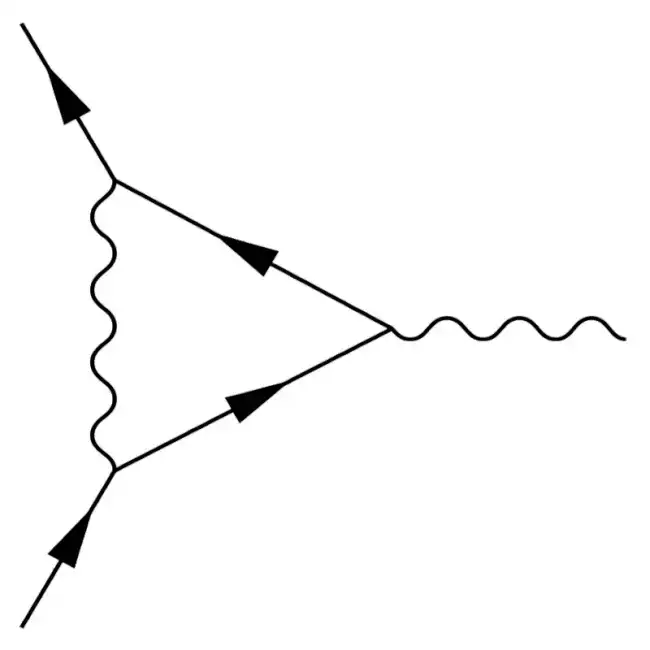## Quantum Field Theory

USOSThis is the mandatory Quantum Field Theory course of the Master in Theoretical Physics at the University of Wrocław. It is tailored towards master and PhD students who are familiar with

There are many good books on the subject, four that I like are

• [PeskSchr] Peskin, Schroeder: An introduction to quantum field theory
• [Ryder] Ryder: Quantum Field Theory
• Weinberg: The Quantum Theory of Fields, Volume 1 & 2
• Zee: Quantum Field Theory in a Nutshell.

We will follow mostly the first one in the lectures. There will be 2 hours of lectures and 2 hours of tutorial each week. Exercises will be posted here a week before the tutorial they are discussed in. Please keep in mind that active participation in the tutorials is important to pass the course. M.Sc. Biplab Mahato will be the assistant for the tutorials. Please fell free to contact him () or me if you have any questions. For information about credits points, please refer to the syllabus (in Polish) or contact me directly.

Important: Students will be assigned to exercise problems by the system described here at the Thursday, 9:00 pm, before the tutorial. Please indicate your preferences by then.

Exam: The exam will be a written exam at the end of the semster. We will discuss a practise exam in the last tutorial to help you in preparing for it.

Additional material for the individual lectures, including the exercises which we discuss in the tutorials, is given below:

1. Reminder of spin 0 and 1/2 fields
Lecture02.03.2023 09:15, notes
Tutorial07.03.2023 11:00, exercise

[PeskSchr] sections one and two

Please note that there are no exercise assignments for the first seminar. Instead, we continue with the lecture.

2. Reminder of spin 1 fields and abelian gauge symmetries
Lecture07.03.2023 09:15, notes
Tutorial14.03.2023 11:15, exercise

[Ryder] sections 3.3 and 4.4

3. Non-abelian gauge symmetries and Lie groups
Lecture14.03.2023 09:15, notes
Tutorial21.03.2023 11:15, exercise

[PeskSchr] section 15 except for 15.3 or alternatively [Ryder] sections 3.5 and 3.6

4. Path integral in quantum mechanics and for the scalar field
Lecture21.03.2023 09:15, notes
Tutorial28.03.2023 11:15, exercise

[PeskSchr] section 9.1

5. Generating functional, interactions and Feynman rules
Lecture28.03.2023 08:15
Tutorial04.04.2023 11:15, exercise

[PeskSchr] section 9.2

6. Path integral for fermions, Grassmann numbers, chiral anomaly (in the exercise)
Lecture04.04.2023 08:15

[PeskSchr] section 9.5

7. Path integral for spin-1 bosons, ghost fields
Lecture18.04.2023 08:15

[PeskSchr] section 9.4

8. One loop effects in QED: field-strength renormalisation and self-energy
Lecture25.04.2023 08:15

[PeskSchr] section 7.1

9. Dimensional regularisation and superficial degree of divergence
Lecture09.05.2023 08:15

Peskin&Schroeder sections 7.5 and 10.1

10. One-loop renoramlised $\phi^4$ theory
Lecture16.05.2023 08:15

[PeskSchr] section 10.2

11. Renormalisation group flow
Lecture23.05.2023 08:15

[PresSchr] section 12.1

12. The Callan-Symanzik equation and $\beta$-functions
Lecture30.05.2023 08:15

[PresSchr] sections 12.2

13. Gravity, quantum gravity, one-loop $\beta$-functions of a two-dimensional $\sigma$-model and string theory
Lecture07.03.2023 09:15
14. Spontaneous symmetry breaking and the Higgs mechanism
Lecture13.06.2023 08:15

[PresSchr] sections 11.1 and 20.1

In the tutorial, we discuss the practise exam.

15. BRST symmetry, physical Hilbert space
Lecture20.06.2023 08:15

[PeskSchr] section 16.2-16.4